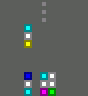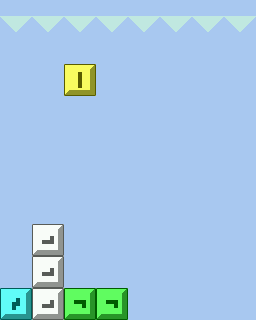-=+=- -=+=- -=+=- -=+=- -=+=- -=+=- -=+=- -=+=- -=+=- -=+=- -=+=- -=+=- -=+=- -=+=- -=+=- -=+=- -=+=- -=+=- -=+=- -=+=- -=+=- -=+=- -=+=- -=+=- -=+=- -=+=- -=+=- -=+=- -=+=- -=+=- (c) WidthPadding Industries 1987 0|68|0 -=+=- -=+=- -=+=- -=+=- -=+=- -=+=- -=+=- -=+=- -=+=- -=+=- -=+=- -=+=- -=+=- -=+=- -=+=- -=+=- -=+=- -=+=- -=+=- -=+=- -=+=- -=+=- -=+=- -=+=- -=+=- -=+=- -=+=- -=+=- -=+=- -=+=-
SoCoder -> Showcase Home -> Arcade

PioCreated : 16 September 2008
Edited : 19 January 2019
System : Windows, Linux
Language : FreeBasic

### Generic Block Game

Configurable falling blocks game

Version 0.7 (Windows 32-bit binary)
Git - source only
ScreenshotsGeneric block game is made in FreeBasic. It is similar to some popular falling blocks games, but following things can be changed:
• Playfield size
• Blocks number and shapes
• Game goals
• Game mechanics (gravity/no gravity)
• Graphics

Variations are made by editing a text file.

Included in this quickly-compiled package are several similar games with falling blocks.

## Latest Update

|edit| December 2018 |edit|
New version of PC game added.
Game can be compiled with FreeBasic.

|edit| January 2019 |edit|
v0.6: dark tiles, resolution and mouse fixes.
v0.7: lighter on CPU usage, temporary highscore, menu fixes